# SSC Worksheet for chapter-8 Circles class 9

## Find SSC Worksheet for chapter-8 Circles class 9

CLASS-9

BOARD: SSC

Mathematic Worksheet - 8

TOPIC: Circles

For other SSC Worksheet for class 9 Mathematic check out main page of Physics Wallah.

1. The radius of a 8√2cm circle is cm find the length of the largest chord of that circle.
2. The radius of a circle with centre P is 25cm and the length the chord is 48cm find the distance of the chord from centre P of the circle.
3. If the length of the largest chord of a circle is 17cm find the radius of a circle.
4. The radius of a circle with centre O is 41 the length of the chord PQ of same circle is 80. Determine the distance of chord PQ from centre O.
5. In figure O is the centre of a circleAB=16cm , seg OM ⊥seg AB, seg ON⊥. seg CD If , OM=6cm then find seg ON.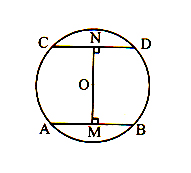6. HI and JK are two parallel chords of a circle which are on opposite side of the centre such that and the distance between them is 7cm then find the radius of the circle.
7. The radius of a circle is 16cm the mid point of chord of a circles lies on the diameter perpendicular to the chord and its distance from one end of the diameter is 3cm; find the ;length of that chord.
8. Find the area of triangle so formed in a circle of radius 40cm, if angle subtended by the chord at the centre of the circle is .
9. In a circle of radius 17cm two parallel chords of the length 30cm and 16cm respectively are drawn on the opposite of the centre then find the distance between them.

## Find SSC Worksheet for chapter-4 congruence of triangles class 9

CLASS-9

BOARD: SSC

Mathematic Worksheet - 4

TOPIC: congruence of triangles

For other SSC Worksheet for class 9 Mathematic check out main page of Physics Wallah.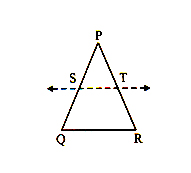• In figure, seg AB≅seg PB, seg BC≅seg BQ , seg BC≅seg CQ, show that also show seg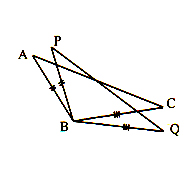• In figure, seg PB≅seg AB≅seg AC≅seg CQ, then find and final all pairs of congruent triangles. Justify your answer.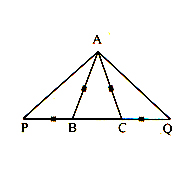• In the figure, ∠DAC≅∠BCA find the missing information which is required and sufficient to prove∠ABC≅∠CBA by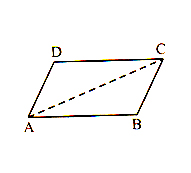1. SAS test
2. SAA test
3. ASA test
• In figure, consider , diagonal MP is perpendicular bisector of diagonal NQ. Then show segMN≅segMQ , andsegPN≅segPQ . Find the pairs of congruent triangles and justify your answer.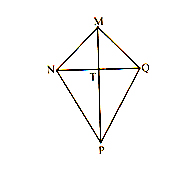• is an isosceles triangle
• seg AN =segAC

segAP , seg BQ, seg CQ are angle bisector of respective angles of .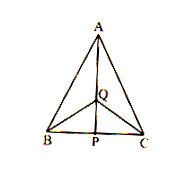1. Find the pairs of congruent triangles
2. Find the triangles which are isosceles
• In ΔABC,∠A=40o, ∠B=80o, find the shortest and the longest side of ΔABC. Justify.
• In figure AB=4cm, AC=6cm, AD=8cm Arrange the angles a, b, c, d in descending order of their measures. Justify.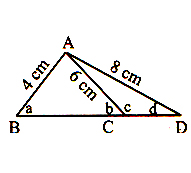• In , sides AB and BC are congruent
1. If A-P-C, then show that congruent sides.
2. If A-C-P , then show that congruent sides.
• In and . What can be the length of the third side PR. Find the minimum and maximum possible length of side PR.